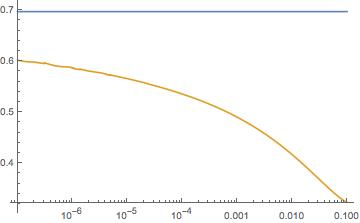# How to get this integral's asymptotics?

Consider the following integral $$\int_0^{\infty}\frac{e^{-x}-1}{x^{2+\frac{A}{\log b-5/6}}}\frac{1}{\log(b/x)-i\pi/2}\,dx$$ where $A>0$ and $b>0$. I am interested in the small $b$ asymptotics of this integral. Any ideas on how to proceed?

• Can you let us know where this problem arises? Aug 8, 2017 at 13:27

The integral in question is \begin{equation*} I:=\int_0^\infty f(x)\,dx, \tag{1} \end{equation*} where \begin{equation*} f(x):=\frac{e^{-x}-1}{x^{2-\varepsilon}}\frac{1}{\ln(b/x)-i\pi/2},\quad \varepsilon:=-\frac{A}{\ln b-5/6}\downarrow0. \end{equation*} Let \begin{equation*} C:=C_\varepsilon:=A-\tfrac56\,\varepsilon, \end{equation*} so that \begin{equation*} b=e^{-C/\varepsilon}. \end{equation*} We have \begin{equation*} \int_{b^{1/2}}^\infty f(x)\,dx \sim\int_{b^{1/2}}^\infty \frac{e^{-x}-1}{x^{2-\varepsilon}}\frac{dx}{\ln(b/x)} =\frac{J_1+J_2}{b^{1-\varepsilon}}, \end{equation*} where \begin{equation*} J_1:=\int_{\varepsilon^{1/2}/b}^\infty \frac{1-e^{-by}}{y^{2-\varepsilon}}\frac{dy}{\ln y} \le\int_{\varepsilon^{1/2}/b}^\infty \frac{dy}{y^{2-\varepsilon}}\frac{1}{\ln(\varepsilon^{1/2}/b)} \ll\varepsilon^{1/2}b^{1-\varepsilon}=o(b^{1-\varepsilon}) \end{equation*} and, with $u=\varepsilon\ln y$, \begin{equation*} J_2:=\int_{b^{-1/2}}^{\varepsilon^{1/2}/b} \frac{1-e^{-by}}{y^{2-\varepsilon}}\frac{dy}{\ln y} \sim b \int_{b^{-1/2}}^{\varepsilon^{1/2}/b} \frac{dy}{y^{1-\varepsilon}}\frac{1}{\ln y} = b \int_{C/2}^{C+\varepsilon\ln(\varepsilon^{1/2})}\frac{du}u\,e^u \sim b(\text{Ei}(A)-\text{Ei}(A/2)). \end{equation*}
It follows that \begin{equation*} \int_{b^{1/2}}^\infty f(x)\,dx \to e^{-A}[\text{Ei}(A)-\text{Ei}(A/2)]. \tag{2} \end{equation*} Also, for \begin{equation*} g(x):=-\frac1{x^{1-\varepsilon}}\frac{1}{\ln(b/x)-i\pi/2}, \end{equation*} we have \begin{equation*} \int_0^{b^{1/2}} |f(x)-g(x)|\,dx \ll\int_0^{b^{1/2}} \frac{x^2}{x^{2-\varepsilon}}\frac{dx}{\pi/2}\to0. \tag{3} \end{equation*} Then, using the substitution $t=\varepsilon\ln(b/x)$, we have \begin{equation*} \int_0^{b^{3/2}} g(x)\,dx\sim -\int_0^{b^{3/2}}\frac{dx}{x^{1-\varepsilon}}\frac{1}{\ln(b/x)} =-e^{-C}\int_{C/2}^\infty \frac{dt}t\,e^{-t}=-e^{-C}\text{E}_1(C/2)\to -e^{-A}\text{E}_1(A/2). \tag{4} \end{equation*} Further, \begin{equation*} \int_{b^{3/2}}^{b^{1/2}} g(x)\,dx= \int_{b^{3/2}}^b g(x)\,dx+\int_b^{b^{1/2}} g(x)\,dx =\int_{b^{1/2}}^1 g(bu)\,b\,du+\int_{b^{1/2}}^1 g(b/u)\,\frac{b\,du}{u^2} =b\times\int_{b^{1/2}}^1 h(u)\,du, \end{equation*} where \begin{equation*} h(u):=g(bu)+g(b/u)/{u^2} =-2i e^{C(1/\varepsilon-1)}e^t\,\frac{2\pi\cosh(\varepsilon t)+4i t \sinh(\varepsilon t)}{\pi^2+4t^2} \end{equation*} and $t:=-\ln u$. (The latter identity is the key: note that $\Re g(x)$, as well as $\Re f(x)$, changes sign at $x=b$.) So, \begin{equation*} \int_{b^{3/2}}^{b^{1/2}} g(x)\,dx= -2i b e^{C(1/\varepsilon-1)}\int_0^{C/(2\varepsilon)}dt\,\frac{2\pi\cosh(\varepsilon t)+4i t \sinh(\varepsilon t)}{\pi^2+4t^2}. \tag{5} \end{equation*} Next, \begin{equation*} \int_0^{C/(2\varepsilon)}dt\,\frac{2\pi\cosh(\varepsilon t)}{\pi^2+4t^2} \to\int_0^\infty dt\,\frac{2\pi}{\pi^2+4t^2}=\frac\pi2 \tag{6} \end{equation*} and \begin{equation*} \int_0^{C/(2\varepsilon)}dt\,\frac{4i t \sinh(\varepsilon t)}{\pi^2+4t^2} =\int_0^{C/2}dz\,\frac{4i z \sinh z}{\varepsilon^2\pi^2+4z^2} \to\int_0^{A/2}dz\,\frac{4i \sinh z}{4z}=i\,\text{shi}\frac A2, \tag{7} \end{equation*} where $\text{shi}$ is the hyperbolic sine integral function. Collecting the pieces (1)--(7), we have \begin{equation*} I\to e^{-A}(\text{Ei}(A)-\text{Ei}(A/2))-e^{-A}\text{E}_1(A/2)-2i e^{-A} (\tfrac\pi2+i\,\text{shi}\tfrac A2) =e^{-A}(\text{Ei}(A)-i\pi), \end{equation*} which is the same expression as the one obtained by Carlo Beenakker.

• very interesting! I note that the function you call ${\rm Ei}$ is what Wikipedia calls ${\rm E}_1$, the relation is ${\rm E}_1(x)=-{\rm Ei}(-x)$ for $x>0$. Your final expression can then be simplified to $I=1/A+e^{-A}[-i\pi+{\rm Ei}\,(A/2)]$. The imaginary part agrees with what I found, but the real part is different: I have ${\rm Re}\,I=e^{-A}{\rm Ei}\,(A)$. For $A=1$ you have ${\rm Re}\,I=1.167$ while I get ${\rm Re}\,I=0.697$. Aug 7, 2017 at 8:34
• @CarloBeenakker : Thank you for your comment. I have followed your suggestions. Aug 7, 2017 at 13:49
• Thank you both Iosif and Carlo for your detailed input. I am sorting out what you wrote Aug 7, 2017 at 13:57
• I made a mistake in estimating $J_2$ and hence in estimating the integral in (2). Now that the mistake is fixed, I get the same expression as Carlo Beenakker's. Aug 7, 2017 at 20:42

$$I(\epsilon,B)=\int_0^{\infty}\frac{e^{-x}-1}{x^{2-\epsilon}}\frac{1}{B-i\pi/2-\log x}\,dx$$ $$\text{with}\;\;\epsilon=-\frac{A}{\log b-5/6}\in(0,1)\;\;\text{and}\;\;B=\log b<0$$

The limit $b\rightarrow 0^+$ corresponds to $\epsilon\rightarrow 0^+$, $B\rightarrow-\infty$ while the product $\epsilon B\rightarrow-A<0$ remains finite. That limit is governed by the small-$x$ behaviour of the integrand in $I(\epsilon,B)$, so we may expand $e^{-x}-1\rightarrow-x+{\cal O}(x^2)$ and calculate $$\lim_{\epsilon\rightarrow 0^+}I(\epsilon,-A/\epsilon)=\lim_{\epsilon\rightarrow 0^+}\int_0^{1}x^{\epsilon-1}\frac{1}{A/\epsilon+\log x+i\pi/2}\,dx=e^{-A}\left[-i\pi+\text{Ei}\,(A)\right],$$ with ${\rm Ei}(x)=-\int_{-x}^\infty t^{-1}e^{-t}\,dt$ the exponential integral function.

The curve below (gold) shows ${\rm Re}\,I$ as a function of $b$ for $A=1$; I was not able to push the numerical integration to smaller $b$, but the numerics is not inconsistent with the analytical result for the asymptote at $e^{-1}\,{\rm Ei}\,(1)=0.697$ (blue horizontal line).• Numeric calculations do not confirm the divergence in the case $A=1$.. Aug 5, 2017 at 17:04
• Could you kindly elaborate your answer because numeric calculations do not confirm the divergence as $b \to 0+$? Aug 5, 2017 at 18:02
• Maple says that $e^{-1}(-i\pi+Ei(1))=0.6971748832- 1.155727350\,i$. This contradicts the numeric calculations from my answer. Aug 5, 2017 at 18:26
• Maple says that $e^{-2}(-i\pi+\text{Ei}(2))=0.6704827096- 0.4251683315\,i$. This also is not in accordance with the numeric calculations from my answer. I'd like to repeat my want about elaboration of your answer. Aug 5, 2017 at 18:35
• @CarloBeenaker This was a tough call but I accepted Iosif's answer because I believe that his careful derivation deserves more credit than the 2 upvotes he has so far. In any case, thank you! Aug 8, 2017 at 11:12

The math experiment done with Maple suggests there exists a limit (of course, depending on $A$) of the integral as $b \to 0+$:

seq(int(eval((exp(-x)-1)/(x^(2+1/(ln(b)-5/6))*(ln(b/x)-I*Pi*(1/2))), b = c),
x = 0 .. infinity, numeric, epsilon = 0.1e-2), c = [0.1e-3, 0.1e-2, 0.1e-1, 0.5e-1, .1]);


$$0.3228143386- 1.088234752\,i, 0.3478772540- 1.118739511\,i, 0.4172578641- 1.160686228\,i, 0.4902769185- 1.178652574\,i, 0.5359078669- 1.181083764\,i.$$

Addition. In the case $A=2$ one obtains

restart; seq(int(eval((exp(-x)-1)/(x^(2+2/(ln(b)-5/6))*(ln(b/x)-I*Pi*(1/2))), b = c),
x = 0 .. infinity, numeric, epsilon = 0.1e-1), c = [0.1e-3, 0.1e-2, 0.1e-1, 0.5e-1]);


$$.6100903815-.5346046841i, .5958857255-.5610607673i, .5824902719-.6031233673i, .6107114031-.6594924349i$$

Addition 2. Here is the Mathematica result in the case $A=1,\,b=0.0001$:

NIntegrate[((Exp[-x] - 1)/(x^(2 + 1/(Log[b] - 5/6))*(Log[b/x] - I*Pi/2))) /.
b -> 0.0001, {x, 0, Infinity}, AccuracyGoal -> 3]


$$0.535836\, -1.18149 i$$

This is in accordance with the above Maple result $0.5359078669- 1.181083764\,i$.

Also

NIntegrate[((Exp[-x] - 1)/(x^(2 + 1/(Log[b] - 5/6))*(Log[b/x] - I*Pi/2))) /.
b -> 0.000001, {x, 0, Infinity}, AccuracyGoal -> 2]


$0.617192 - 1.17718 i.$

• Remark. The integral is calculated for $A=1, b \in \{0.0001, 0.001, 0.01, 0.05\}$ with the relative error $0.001$ and for $A=2, b \in \{ 0.0001, 0.001,0,01 ,0.05\}$ with the relative error $0.01$. Aug 5, 2017 at 17:55
• notice that $b$ appears as $\log b$, so the convergence in $b$ is very slow, even for $b=0.0001$ you only have $-1/\log b\approx 0.1$, so not very small. Aug 6, 2017 at 11:11
• Coud you kindly base your statement? No remainder estimate is given in your answer. The limit is taken over $b$, not over $\log b$. Aug 6, 2017 at 11:21
• I prefer arguments over unbased sentences e.g. "so it is reasonable to assume ". How about you? Aug 6, 2017 at 11:26
• It seems you are right because Mathematica outputs $0.686336\, -1.17138 i$ for $A=1, b=0.00000001$. Aug 6, 2017 at 11:35